# 4.1 Linear functions  (Page 9/27)

 Page 9 / 27

## Vertical stretch or compression

In the equation $\text{\hspace{0.17em}}f\left(x\right)=mx,$ the $\text{\hspace{0.17em}}m\text{\hspace{0.17em}}$ is acting as the vertical stretch    or compression of the identity function. When $\text{\hspace{0.17em}}m\text{\hspace{0.17em}}$ is negative, there is also a vertical reflection of the graph. Notice in [link] that multiplying the equation of $\text{\hspace{0.17em}}f\left(x\right)=x\text{\hspace{0.17em}}$ by $\text{\hspace{0.17em}}m\text{\hspace{0.17em}}$ stretches the graph of $\text{\hspace{0.17em}}f\text{\hspace{0.17em}}$ by a factor of $\text{\hspace{0.17em}}m\text{\hspace{0.17em}}$ units if $\text{\hspace{0.17em}}m>\text{1}\text{\hspace{0.17em}}$ and compresses the graph of $\text{\hspace{0.17em}}f\text{\hspace{0.17em}}$ by a factor of $\text{\hspace{0.17em}}m\text{\hspace{0.17em}}$ units if $\text{\hspace{0.17em}}0 This means the larger the absolute value of $\text{\hspace{0.17em}}m,\text{\hspace{0.17em}}$ the steeper the slope.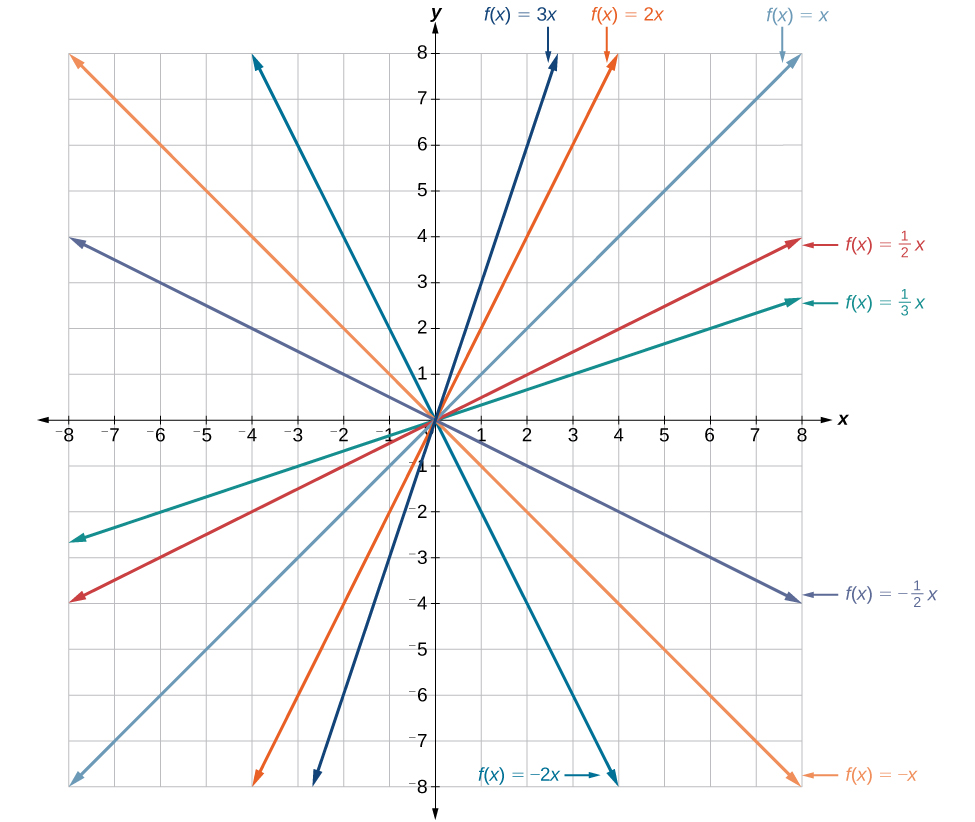Vertical stretches and compressions and reflections on the function   f ( x ) = x

## Vertical shift

In $\text{\hspace{0.17em}}f\left(x\right)=mx+b,$ the $\text{\hspace{0.17em}}b\text{\hspace{0.17em}}$ acts as the vertical shift    , moving the graph up and down without affecting the slope of the line. Notice in [link] that adding a value of $\text{\hspace{0.17em}}b\text{\hspace{0.17em}}$ to the equation of $\text{\hspace{0.17em}}f\left(x\right)=x\text{\hspace{0.17em}}$ shifts the graph of $\text{\hspace{0.17em}}f\text{\hspace{0.17em}}$ a total of $\text{\hspace{0.17em}}b\text{\hspace{0.17em}}$ units up if $\text{\hspace{0.17em}}b\text{\hspace{0.17em}}$ is positive and $\text{\hspace{0.17em}}|b|\text{\hspace{0.17em}}$ units down if $\text{\hspace{0.17em}}b\text{\hspace{0.17em}}$ is negative.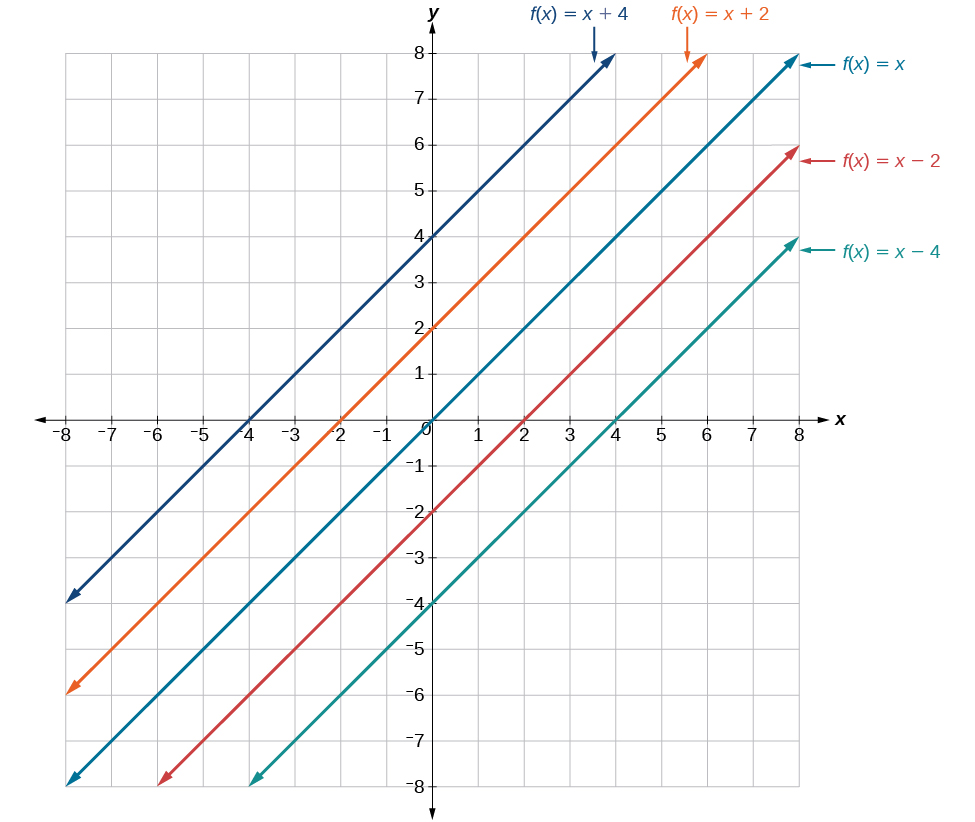This graph illustrates vertical shifts of the function f ( x ) = x .

Using vertical stretches or compressions along with vertical shifts is another way to look at identifying different types of linear functions. Although this may not be the easiest way to graph this type of function, it is still important to practice each method.

Given the equation of a linear function, use transformations to graph the linear function in the form $\text{\hspace{0.17em}}f\left(x\right)=mx+b.$

1. Graph $\text{\hspace{0.17em}}f\left(x\right)=x.$
2. Vertically stretch or compress the graph by a factor $\text{\hspace{0.17em}}m.$
3. Shift the graph up or down $\text{\hspace{0.17em}}b\text{\hspace{0.17em}}$ units.

## Graphing by using transformations

Graph $\text{\hspace{0.17em}}f\left(x\right)=\frac{1}{2}x-3\text{\hspace{0.17em}}$ using transformations.

The equation for the function shows that $\text{\hspace{0.17em}}m=\frac{1}{2}\text{\hspace{0.17em}}$ so the identity function is vertically compressed by $\text{\hspace{0.17em}}\frac{1}{2}.\text{\hspace{0.17em}}$ The equation for the function also shows that $\text{\hspace{0.17em}}b=-3\text{\hspace{0.17em}}$ so the identity function is vertically shifted down 3 units. First, graph the identity function, and show the vertical compression as in [link] .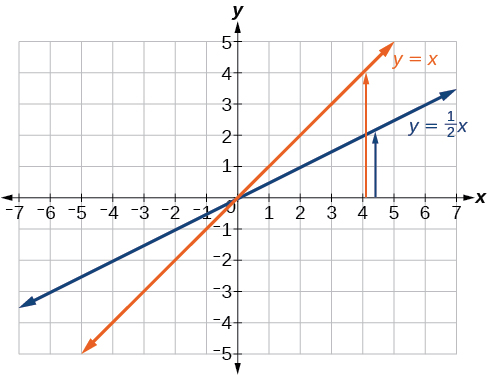The function,   y = x , compressed by a factor of   1 2

Then show the vertical shift as in [link] .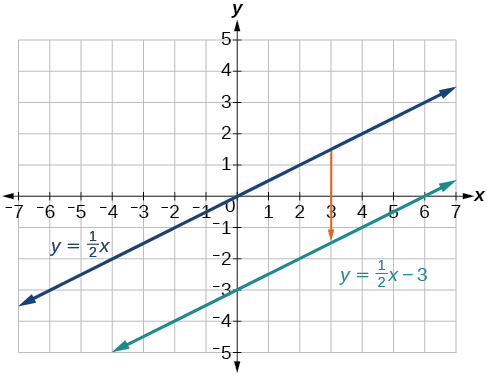The function   y = 1 2 x , shifted down 3 units

Graph $\text{\hspace{0.17em}}f\left(x\right)=4+2x\text{\hspace{0.17em}}$ using transformations.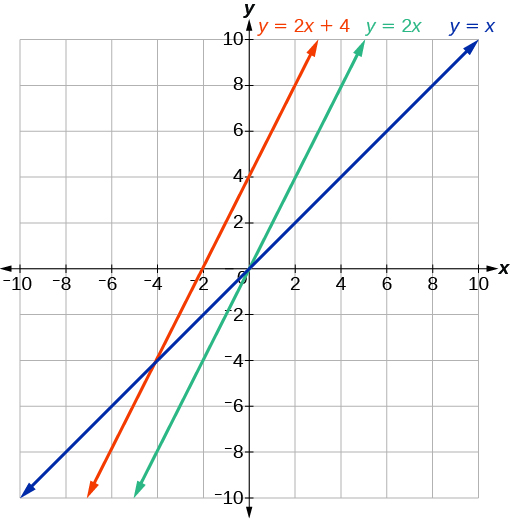In [link] , could we have sketched the graph by reversing the order of the transformations?

No. The order of the transformations follows the order of operations. When the function is evaluated at a given input, the corresponding output is calculated by following the order of operations. This is why we performed the compression first. For example, following the order: Let the input be 2.

$\begin{array}{ccc}\hfill f\left(2\right)& =& \frac{1}{2}\left(2\right)-3\hfill \\ & =& 1-3\hfill \\ & =& -2\hfill \end{array}$

## Writing the equation for a function from the graph of a line

Earlier, we wrote the equation for a linear function from a graph. Now we can extend what we know about graphing linear functions to analyze graphs a little more closely. Begin by taking a look at [link] . We can see right away that the graph crosses the y -axis at the point $\text{\hspace{0.17em}}\left(0,\text{4}\right)\text{\hspace{0.17em}}$ so this is the y -intercept.

Then we can calculate the slope by finding the rise and run. We can choose any two points, but let’s look at the point $\text{\hspace{0.17em}}\left(–2,0\right).\text{\hspace{0.17em}}$ To get from this point to the y- intercept, we must move up 4 units (rise) and to the right 2 units (run). So the slope must be

$m=\frac{\text{rise}}{\text{run}}=\frac{4}{2}=2$

Substituting the slope and y- intercept into the slope-intercept form of a line gives

root under 3-root under 2 by 5 y square
The sum of the first n terms of a certain series is 2^n-1, Show that , this series is Geometric and Find the formula of the n^th
cosA\1+sinA=secA-tanA
why two x + seven is equal to nineteen.
The numbers cannot be combined with the x
Othman
2x + 7 =19
humberto
2x +7=19. 2x=19 - 7 2x=12 x=6
Yvonne
because x is 6
SAIDI
what is the best practice that will address the issue on this topic? anyone who can help me. i'm working on my action research.
simplify each radical by removing as many factors as possible (a) √75
how is infinity bidder from undefined?
what is the value of x in 4x-2+3
give the complete question
Shanky
4x=3-2 4x=1 x=1+4 x=5 5x
Olaiya
hi can you give another equation I'd like to solve it
Daniel
what is the value of x in 4x-2+3
Olaiya
if 4x-2+3 = 0 then 4x = 2-3 4x = -1 x = -(1÷4) is the answer.
Jacob
4x-2+3 4x=-3+2 4×=-1 4×/4=-1/4
LUTHO
then x=-1/4
LUTHO
4x-2+3 4x=-3+2 4x=-1 4x÷4=-1÷4 x=-1÷4
LUTHO
A research student is working with a culture of bacteria that doubles in size every twenty minutes. The initial population count was  1350  bacteria. Rounding to five significant digits, write an exponential equation representing this situation. To the nearest whole number, what is the population size after  3  hours?
v=lbh calculate the volume if i.l=5cm, b=2cm ,h=3cm
Need help with math
Peya
can you help me on this topic of Geometry if l help you
litshani
( cosec Q _ cot Q ) whole spuare = 1_cosQ / 1+cosQ
A guy wire for a suspension bridge runs from the ground diagonally to the top of the closest pylon to make a triangle. We can use the Pythagorean Theorem to find the length of guy wire needed. The square of the distance between the wire on the ground and the pylon on the ground is 90,000 feet. The square of the height of the pylon is 160,000 feet. So, the length of the guy wire can be found by evaluating √(90000+160000). What is the length of the guy wire?
the indicated sum of a sequence is known as
how do I attempted a trig number as a starter
cos 18 ____ sin 72 evaluateByByBy Stephen VoronBy Brooke DelaneyBy Nick SwainByBy Sarah WarrenBy Brooke DelaneyBy David CoreyBy Anonymous UserBy JavaChamp TeamBy Anh Dao